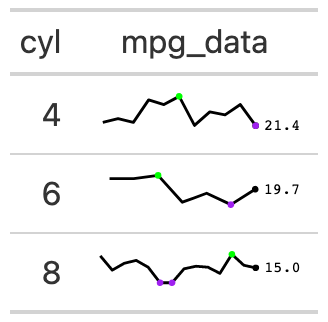The gt_sparkline function takes an existing gt_tbl object and adds sparklines via the ggplot2. This is a wrapper around gt::text_transform() + ggplot2 with the necessary boilerplate already applied.

## Usage

gt_sparkline(
gt_object,
column,
type = "sparkline",
width = 30,
line_color = "black",
range_colors = c("red", "blue"),
fill_color = "grey",
bw = NULL,
trim = FALSE,
same_limit = TRUE,
label = TRUE
)

## Arguments

gt_object

An existing gt table object of class gt_tbl

column

The column wherein the sparkline plot should replace existing data. Note that the data must be represented as a list of numeric values ahead of time.

type

A string indicating the type of plot to generate, accepts "sparkline", "histogram" or "density".

width

A number indicating the width of the plot in mm at a DPI of 25.4, defaults to 30

line_color

Color for the line, defaults to "black". Accepts a named color (eg 'blue') or a hex color.

range_colors

A vector of two valid color names or hex codes, the first color represents the min values and the second color represents the highest point per plot. Defaults to c("blue", "blue"). Accepts a named color (eg 'blue') or a hex color like "#fafafa".

fill_color

Color for the fill of histograms/density plots, defaults to "grey". Accepts a named color (eg 'blue') or a hex color.

bw

The bandwidth or binwidth, passed to density() or ggplot2::geom_histogram(). If type = "density", then bw is passed to the bw argument, if type = "histogram", then bw is passed to the binwidth argument.

trim

A logical indicating whether to trim the values in type = "density" to a slight expansion beyond the observable range. Can help with long tails in density plots.

same_limit

A logical indicating that the plots will use the same axis range (TRUE) or have individual axis ranges (FALSE).

label

A logical indicating whether the sparkline will have a numeric label at the end of the plot.

## Value

An object of class gt_tbl.

## Figures## Function ID

1-4

Other Plotting: gt_plt_bar_pct(), gt_plt_bar_stack(), gt_plt_bar(), gt_plt_dist(), gt_plt_percentile(), gt_plt_point(), gt_plt_sparkline(), gt_plt_winloss()

## Examples

 library(gt)
gt_sparkline_tab <- mtcars %>%
dplyr::group_by(cyl) %>%
# must end up with list of data for each row in the input dataframe
dplyr::summarize(mpg_data = list(mpg), .groups = "drop") %>%
gt() %>%
gt_sparkline(mpg_data)